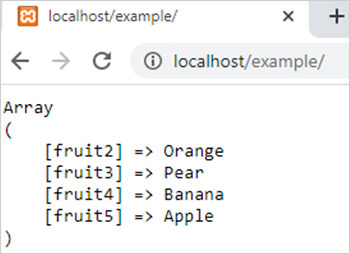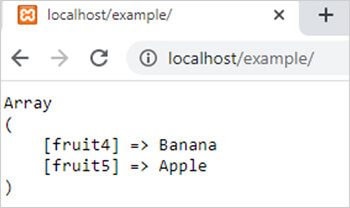array_diff_assoc() function in php

array_diff_assoc() function in php

0 1136

array_diff_assoc() function of PHP is used to calculate the difference of two or more associative arrays means it compares the keys and values of two or more arrays and return the items of first array which is different. It is an inbuilt function of PHP.

Syntax

array_diff_assoc(\$arr1,\$arr2,.....,\$arrN);

Here

\$arr1,\$arr2 and \$arrN are associative arrays.

Example 1:

<?php
\$arr1=array("fruit1"=>"Mango","fruit2"=>"Orange","fruit3"=>"Pear","fruit4"=>"Banana","fruit5"=>"Apple");
\$arr2=array("fruit1"=>"Mango","fruit6"=>"Banana");
\$new_arr=array_diff_assoc(\$arr1,\$arr2);
echo "<pre>";
print_r(\$new_arr);
?>

Output:Example 2:

<?php
\$arr1=array("fruit1"=>"Mango","fruit2"=>"Orange","fruit3"=>"Pear","fruit4"=>"Bana  na","fruit5"=>"Apple");
\$arr2=array("fruit1"=>"Mango","fruit6"=>"Banana");
\$arr3=array("fruit2"=>"Orange","fruit3"=>"Pear");
\$new_arr=array_diff_assoc(\$arr1,\$arr2,\$arr3);
echo "<pre>";
print_r(\$new_arr);
?>

Output:Share: